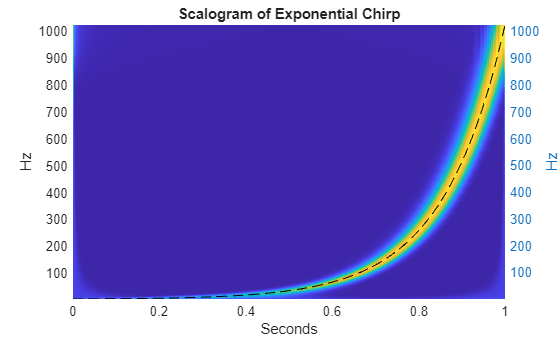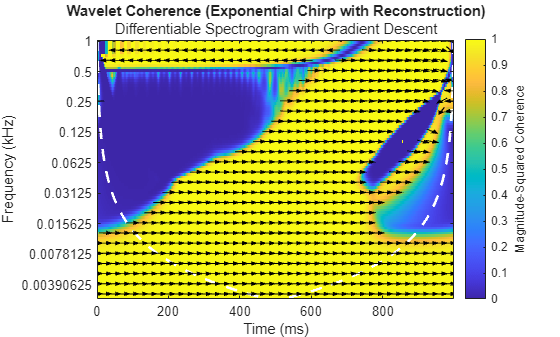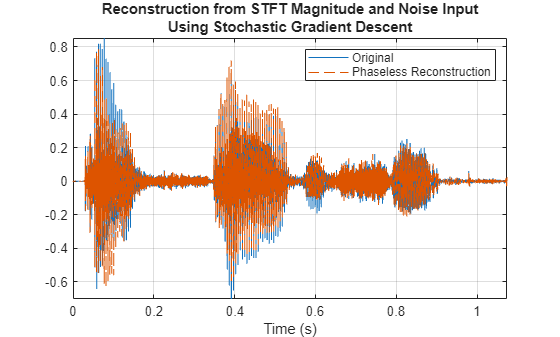# Signal Recovery with Differentiable Scalograms and Spectrograms

This example shows how to use differentiable scalograms and spectrograms to recover a time-domain signal. The use of differentiable time-frequency transforms allows you to obtain an approximation of the original signal without the need for phase information or the need to explicitly invert the time-frequency transform. We demonstrate this technique on synthetic data and on a speech signal. Additionally, the gradient-descent based technique using differentiable spectrograms is compared against the Griffin-Lim algorithm.

### Background

In a number of applications, the phase information in a time-frequency representation is discarded in favor of the magnitudes. There are a number of reasons for this. One reason is simply that the complex-valued time-frequency representations containing phase information are difficult to plot and interpret. This may lead people to retain only the magnitudes. In other applications, the required signal processing is optimally done by modifying the magnitudes of a time-frequency representation. This is most frequently done in speech processing where the underlying time-frequency representation is usually the short-time Fourier transform. In the latter case, the original complex-valued time-frequency representation no longer corresponds to the modified magnitude representation.

In these applications, it still may be useful or even necessary to recover an approximation of the original signal. The techniques for doing this are referred to as phase retrieval. Phase retrieval is, in general, ill-posed and prior iterative methods suffer from the non-convexity of the formulation and therefore convergence to an optimal solution is impossible to guarantee.

With the introduction of automatic differentiation and differentiable signal processing, we can now use gradient descent with the usual convex loss functions to perform phase retrieval.

### Synthetic Signal — Exponential Chirp

In this first example, we create a chirp signal with an exponentially increasing carrier frequency. The chirp is created by the helper function `helperEchirp`. The source code is listed at the end of the example. This synthetic signal is challenging for a signal recovery, or phase retrieval, algorithm due to how rapidly the instantaneous frequency increases over time. The code for creating the exponential chirp is due to .

```[sig,t] = helperEchirp(2048); plot(t,sig) grid on title('Exponential Chirp') xlabel('Seconds') ylabel('Amplitude')```Obtain the scalogram of the chirp and plot the scalogram along with the instantaneous frequency of the chirp. Here the scalogram is plotted using a linear scaling on the frequency (scale) axis to clearly show the exponential nature of the chirp.

```[fmin,fmax] = cwtfreqbounds(2048,Cutoff=100,wavelet='amor'); [cfs,f,~,~,scalcfs] = cwt(sig,FrequencyLimits=[fmin fmax],extend=false); figure t = linspace(0,1,length(sig)); surf(t,f,abs(cfs)) ylabel('Hz') shading interp view(0,90) yyaxis right plot(t,1024.^t./2048,'k--') axis tight title('Scalogram of Exponential Chirp') ylabel('Hz') xlabel('Seconds')```Now, use a differentiable scalogram to perform phase retrieval. Throughout the example, the helper object, `helperPhaseRetrieval`, is used to perform phase retrieval for both the scalogram and spectrogram. By default, `helperPhaseRetrieval` pads the signal symmetrically with 10 samples at the beginning and 10 samples at the end to compensate for edge effects.

First, create an object configured for the scalogram and obtain the scalogram of the chirp signal. Show that the scalogram contains only real-valued data.

```pr = helperPhaseRetrieval(Method='scalogram',wavelet="morse", ... IncludeLowpass=true); sc = obtainTFR(pr,sig); isreal(sc)```
```ans = logical 1 ```

The scalogram is also a `dlarray`, which allows us to record operations performed on it for automatic differentiation.

### Signal recovery using gradient descent

Here we recover an approximation to the original signal using the magnitude scalogram and gradient descent. The helper function `retrievePhase` does this by the following procedure:

1. Initialize random noise the same length as the input signal.

2. Obtain the scalogram of the noise. Measure the mean squared error (MSE) between the scalogram of the target signal and the scalogram of the noise.

3. Use gradient descent with an Adam optimizer to update the noise signal based on the MSE loss between the target scalogram and the scalogram of then noise.

The above procedure is detailed in . At the end of the gradient descent procedure, determine how the noise has converged to a reconstruction of the original signal.

Inside of `retrievePhase`, a noise signal is initialized as a starting point. Here we compare a representative noise exactly like the one used to initiate the gradient descent procedure. Compare the initial noise with the original chirp signal.

```rng default x = dlarray(randn(size(sig)),'CBT'); x = x./max(abs(x),[],3); figure plot(t,squeeze(extractdata(x)),'linewidth',0.5) hold on plot(t,sig,'k',linewidth=2) legend('Random','Chirp signal') title('Random Noise Initialization with Exponential Chirp') axis tight hold off ylim([-1.5 1.5]) xlabel('Seconds')```Use gradient descent and the differentiable scalogram to recover an approximation to the original signal.

`xrec = retrievePhase(pr,sc);`

After 300 iterations of gradient descent, the noise signal is modified to closely approximate the chirp signal. Plot the result of the phase retrieval. Note that phase retrieval for real-valued signals is only defined up to a sign change. Accordingly, the result scaled by 1 or -1 may provide a better result.

```figure plot(t,[xrec sig]) grid on xlabel('Seconds') ylabel('Amplitude') legend('Phase Reconstruction','Original Signal') title('Phase retrieval-- Using Scalogram')```Obtain the scalogram of the reconstructed signal and compare its phase at selected center frequencies (CF) with the phase of the original. The phase is compared by plotting the real and imaginary parts of the continuous wavelet transform (CWT) coefficients separately.

```cfsR = cwt(xrec,FrequencyLimits=[fmin fmax]); figure tiledlayout(3,2); nexttile(1) plot(t,[real(cfs(30,:))' real(cfsR(30,:))']) grid on fstr = sprintf('%2.2f',f(30)); title({'Scalogram -- Real Part'; ['CF ',fstr, ' cycles/sample']}) xlim([0.63 0.79]) nexttile(2) plot(t,[imag(cfs(30,:))' imag(cfsR(30,:))']) grid on title({'Scalogram -- Imaginary Part'; ['CF ',fstr, ' cycles/sample']}) xlim([0.63 0.79]) nexttile(3) plot(t,[real(cfs(40,:))' real(cfsR(40,:))']) grid on fstr = sprintf('%2.2f',f(40)); title({'Scalogram -- Real Part'; ['CF ',fstr, ' cycles/sample']}) xlim([0.48 0.76]) nexttile(4) plot(t,[imag(cfs(40,:))' imag(cfsR(40,:))']) grid on title({'Scalogram -- Imaginary Part'; ['CF ',fstr, ' cycles/sample']}) xlim([0.48 0.76]) nexttile(5) plot(t,[real(cfs(50,:))' real(cfsR(50,:))']) grid on fstr = sprintf('%2.2f',f(50)); title({'Scalogram -- Real Part'; ['CF ',fstr, ' cycles/sample']}) xlim([0.4 0.62]) nexttile(6) plot(t,[imag(cfs(50,:))' imag(cfsR(50,:))']) grid on title({'Scalogram -- Imaginary Part'; ['CF ',fstr, ' cycles/sample']}) xlim([0.4 0.62])```The phase agreement for the selected center frequencies is quite good. In fact, if we look at the wavelet coherence between the original signal and its reconstructed version using the gradient-descent based phase retrieval, the overall agreement is quite strong.

```figure wcoherence(sig,xrec,FrequencyLimits=[0 1/2],PhaseDisplayThreshold=0.7) titlestr = get(gca,'Title'); titlestr.String = ... {'Wavelet Coherence -- Exponential Chirp with reconstruction'; ... 'Differentiable Scalogram with Gradient Descent'};```Note the arrows representing the phase coherence between the original signal the reconstruction are oriented at zero degrees, or $2\pi$, radians indicating perfect phase agreement. The area near of low phase agreement near the beginning of the signal in the high frequencies should be interpreted with caution. The instantaneous frequency of the chirp signal is relatively low initially and therefore there is no energy in the original signal at high frequencies at that point.

Repeat the same process using the spectrogram as the time-frequency representation instead of the scalogram. Here a Hamming window of 256 samples with an overlap of 254 samples is used. For the spectrogram increase the padding to 30 samples, 15 pre-padded and 15 post-padded.

```pr = helperPhaseRetrieval(Method='spectrogram',Window=hann(256,'periodic'), ... OverlapLength=254,Padding=30); sp = obtainTFR(pr,sig); xrec = retrievePhase(pr,sp); figure plot(t,[-xrec sig]) grid on legend('Phase Reconstruction','Original Signal') title('Phase retrieval -- Using Spectrogram')```The recovery from the spectrogram in this case is also quite good. Use wavelet coherence again to look at the time-varying phase coherence between the original signal and the output of phase retrieval approach using gradient descent with the differentiable spectrogram.

```figure wcoherence(sig,-xrec,FrequencyLimits=[0 1/2],PhaseDisplayThreshold=0.7) titlestr = get(gca,'Title'); titlestr.String = ... {'Wavelet Coherence -- Exponential Chirp with reconstruction'; ... 'Differentiable Spectrogram with Gradient Descent'};```Similar to the scalogram, the phase coherence between the two signals is quite strong.

### Comparison with Griffin-Lim

Let us attempt the same phase retrieval with an approach that does not depend on differentiable signal processing and backpropagation. Here we use the Griffin-Lim algorithm which is a commonly used iterative technique for phase retrieval. However, unlike the approach using gradient descent and backpropagation, Griffin-Lim requires the inverse short-time Fourier transform at each iteration. Like many phase-retrieval techniques, Griffin-Lim can exhibit edge effects. To attempt to mitigate these effects, obtain the Griffin-Lim result both without and without signal extension.

```S = stft(sig,Window= hamming(256),OverlapLength=254,FrequencyRange='onesided'); xrecGLNoPad = stftmag2sig(abs(S),256,window=hamming(256), ... OverlapLength=254,FrequencyRange='onesided'); figure plot(t,[-xrecGLNoPad sig]) grid on legend('Phase Reconstruction','Original Signal') title('Phase retrieval -- Using Griffin-Lim')```Note that the Griffin-Lim approach exhibits some significant artifacts at the beginning of the signal and again around 0.86 seconds. Extend the original signal symmetrically at the ends to try and mitigate these effects. This is the same padding done in the `helperPhaseRetrieval` object.

```xpad = padsequences({sig},1,'direction','both','length',2048+30, ... 'paddingvalue','symmetric'); S = stft(xpad,Window= hamming(256),OverlapLength=254,FrequencyRange='onesided'); xrecGLpad = stftmag2sig(abs(S),256,window=hamming(256),OverlapLength=254, ... FrequencyRange='onesided'); xrecGLpad = xrecGLpad(16:end-15); figure plot(t,[-xrecGLpad sig]) grid on legend('Phase Reconstruction','Original Signal') title('Phase retrieval -- Using Griffin-Lim')```Symmetrically extending the signal does not mitigate the artifacts and it fact exacerbates them at the end of the signal. For completeness, you can attempt to initialize the Griffin-Lim algorithm more closely to the gradient-descent technique by using the optional `InitializePhaseMethod` input and setting its value to `'random'`.

```S = stft(sig,Window= hamming(256),OverlapLength=254,FrequencyRange='onesided'); xrecGLNoPadRandom = stftmag2sig(abs(S),256,window=hamming(256), ... OverlapLength=254,FrequencyRange='onesided',InitializePhaseMethod="random"); figure plot(t,[-xrecGLNoPadRandom sig]) grid on legend('Phase Reconstruction','Original Signal') title({'Phase retrieval -- Using Griffin-Lim'; 'Random Noise Initialization'})```This appears to result in a worse approximation of the original signal. Accordingly, use the original reconstruction from the STFT magnitudes using the Griffin-Lim algorithm and examine the wavelet coherence between the original signal and the reconstruction.

```figure wcoherence(sig,-xrecGLNoPad,FrequencyLimits=[0 1/2],PhaseDisplayThreshold=0.7) titlstr = get(gca,'Title'); titlstr.String = ... {'Wavelet Coherence -- Exponential Chirp with reconstruction'; ... 'Griffin-Lim Agorithm using Spectrograms'};```In this instance, the differentiable phase-retrieval technique does a significantly better job at reconstructing the original phase than the Griffin-Lim algorithm. You can verify this numerically by examining the relative L2-norm error between the original signal and the approximations.

`RelativeL2DiffGD = norm(sig-(-xrec),2)/norm(sig,2)`
```RelativeL2DiffGD = 0.6392 ```
`RelativeL2DiffGL = norm(sig-(-xrecGLNoPad))/norm(sig,2)`
```RelativeL2DiffGL = 1.2106 ```

### Speech Signal

As mentioned in the introduction, a common application of signal recovery from magnitude time-frequency transforms is in speech. Here we apply differentiable signal processing and gradient descent to speech.

Load and play a speech sample of a speaker saying "I saw the sheep". The original data is sampled at 22050 Hz, resample the data at 1/2 the original rate.

```load wavsheep.mat sheep = resample(sheep,1,2); fs = fs/2; soundsc(sheep,fs)```

As usual, a random noise input is used as the starting point for the phase retrieval algorithm. Here we plot a representative noise sample to show how different the characteristics of the initial noise are from the speech signal.

```x = randn(size(sheep)); x = x./max(abs(x),[],3); t = linspace(0,length(sheep)*1/fs-1/fs,length(sheep)); figure plot(t,[x sheep]) legend('Random','I saw the sheep') ylim([-1.5, 1.5]) xlim([0 length(sheep)*1/fs-1/fs]) xlabel('Seconds') ylabel('Amplitude') title('Random Noise Input and Target Speech Signal')```Recover an approximation of the speech signal from the magnitude spectrogram using gradient descent. Use a Hamming window with 256 samples and overlap the windows by 75% of the window length, or 192 samples. Use 200 iterations of gradient descent.

```win = hamming(256); overlap = 0.75*256; pr = helperPhaseRetrieval(Method='spectrogram',window=win,OverlapLength=overlap); sc = obtainTFR(pr,sheep); [xrec,x_recon] = retrievePhase(pr,sc,NumIterations=200);```

Plot the result. Note that what started as just random noise has converged to a good approximation of the speech signal. There is always a phase ambiguity between a value, V, and its negative, -V. So you can also try plotting the negative.

```figure plot(t,[sheep -xrec]) title({'Reconstruction from magnitude STFT and noise input'; ... 'using stochastic gradient descent'}) legend('Original','Phaseless reconstruction') axis tight grid on```Play the original waveform and the reconstruction. Pause 3 seconds between playbacks.

```soundsc(sheep,fs) pause(3) soundsc(xrec,fs)```

The perceptual quality of the reconstruction is quite good. Having retained all the iterations of the gradient descent algorithm in the variable, `x_recon`, you can verify that already at the 50-th iteration of the algorithm, human speech starts to differentiate from the noise. By the 125-th iteration, the perceptual quality of the reconstruction is nearly indistinguishable from the original speech sample.

```soundsc(x_recon(50,:),fs) pause(3) soundsc(x_recon(125,:),fs)```

Repeat the same procedure using the magnitude CWT as an input. Because the CWT is more computationally expensive than the STFT, the time for the gradient descent algorithm to complete 200 iterations is significantly longer. However, another advantage of using differentiable signal processing tools built on `dlarray` is that you can also leverage GPU acceleration if you have a suitable GPU. In this case, use an NVIDIA Titan V with a compute capability of 7.0 accelerated the signal recovery procedure by a factor of 3. You can toggle back on forth between `None` and `GPU` to determine if your GPU results in a reduction in computation time.

```accel = "None"; pr = helperPhaseRetrieval(Method='scalogram'); sc = obtainTFR(pr,sheep); [xrec,x_recon] = retrievePhase(pr,sc,acceleration=accel,NumIterations=200);```

Plot the result. Similar to the case with the magnitude STFT, what began as random noise has converged to a good approximation of the speech signal. There is always a phase ambiguity between a value, V and its negative -V. So you can also try plotting the negative.

```figure plot(t,[sheep xrec]) title({'Reconstruction from scalogram and noise input'; ... 'using stochastic gradient descent'}) legend('Original','Phaseless reconstruction') axis tight grid on```Play the original waveform and the reconstruction. Pause 3 seconds between playbacks. The scalogram technique also produces an approximation which is perceptually equivalent to the original.

```soundsc(sheep,fs) pause(3) soundsc(-xrec,fs)```

Similar to what was done with the magnitude STFT, all iterations of the gradient descent algorithm were retained in the variable, `x_recon`. You can verify that already on just the 50-th iteration of the algorithm, human speech starts to differentiate from the noise. By the 125-th iteration, the perceptual quality of the reconstruction is nearly indistinguishable from the original speech sample.

```soundsc(x_recon(50,:),fs) pause(3) soundsc(x_recon(125,:),fs)```

### Conclusion

In this example, we showed how differentiable signal processing algorithms can be used with gradient descent to recover signal approximations from magnitude time-frequency representations. One advantage of the differentiable spectrogram or scalogram approach illustrated here is that no inverse transform is required. There are a number of refinements that can be made to the algorithm shown here to improve its robustness. These include using different optimizers, implementing a piecewise change in the learning rate, and even switching over to L1 loss when the overall loss becomes small. The latter technique is demonstrated in . Another option is to initialize the algorithm with an input signal which is closer to the target signal than the white noise used in this example.

### References

 Muradeli, John. “Stack Exchange Answers.” Inverting a Scalogram, 3 Oct. 2021, https://dsp.stackexchange.com/questions/78530/inverting-a-scalogram.

### Appendix -- Helper Functions

Helper function to create chirp with exponentially increasing center frequency.

```function [sig,t] = helperEchirp(N) fmax = N/2; t = linspace(0,1,N); a = 1; b = fmax; sig = cos(2*pi*a/log(b)*b.^t); sig = sig(:); end```